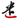← HOME

Know a little byte of programming.

# JavaScrit Coercion

July 29, 2019

``[] == ![]``

### 一. 关于 `==`

1. If Type(x) is the same as Type(y), then

1. Return the result of performing Strict Equality Comparison x === y.
2. If x is null and y is undefined, return true.
3. If x is undefined and y is null, return true.
4. If Type(x) is Number and Type(y) is String, return the result of the comparison x == ! ToNumber(y).
5. If Type(x) is String and Type(y) is Number, return the result of the comparison ! ToNumber(x) == y.
6. If Type(x) is Boolean, return the result of the comparison ! ToNumber(x) == y.
7. If Type(y) is Boolean, return the result of the comparison x == ! ToNumber(y).
8. If Type(x) is either String, Number, or Symbol and Type(y) is Object, return the result of the comparison x == ToPrimitive(y).
9. If Type(x) is Object and Type(y) is either String, Number, or Symbol, return the result of the comparison ToPrimitive(x) == y.
10. Return false.

ToNumber 和 ToPrimitive 参考下一节。

a. 步骤 1 表示如果 x 与 y 类型相同，结果等同与 `x === y`;

b. 步骤 2 与步骤 3 表示 `null == undefined` 结果为 true；

c. 步骤 4 与步骤 5 表示如果 x 与 y 一方是 Number，另一方是 String，对 String 执行 ToNumber，再进行比较.

d. 步骤 6 与步骤 7 表示如果 x 与 y 一方是 Boolean，则对 这一方 执行 ToNumber，再进行比较.

e. 步骤 8 与步骤 9 表示如果 x 与 y 一方是 String, Number 或 Symbol，另一方是 Object，则对 Object 执行 ToPrimitive .

f. 步骤 10 表示如果能执行到这一步，直接返回 false。

1. `null == 3` 可以到达步骤 10.
2. 各个步骤之间是 if … else if … 的关系，原文中有 return，解释时偷懒没加上。
3. 从步骤 6, 7 可以看出，双等号 `==` 偏向 Number 的比较。如果类型不同，一般转换结果是把两方都变成 Number 类型。

### 二. 转换操作

JavaScript 中的类型转换会借助一类抽象操作（Abstract Operation），类似于抽象函数，可以接受参数。

#### 1: ToBoolean(argument)

argument 类型 转换结果
Boolean 无需转换
Undefined false
Null false
Number +0, -0, NaN 返回 false，其余返回 true
String 空字符串（长度为 0) 返回 false, 否则返回 true
Symbol true
Object true

#### 2: ToNumber(argument)

argument 类型 转换结果
Number 无需转换
Undefined NaN
Null +0
Boolean true 转为 1，false 转为 +0
String 【见下文】
Symbol 抛出 TypeError 异常
Object 先调用 ToPrimitive(argument, hint Number), 再将结果传入 ToNumber

String 转为 Number 有一套单独的语法，简单理解为先去除首尾空白字符，然后：

1. 如果结果是空字符串，转为 0；
2. 如果结果是数字形式，则转为对应数字；
3. 其它情况都转为 NaN。
``````Number("") // => 0
Number("  \n \t0.  ") // => 0
Number("\t 10.35 ") // => 10.35
Number("\t 1.0.2 ") // => NaN
Number("1.x") // => NaN``````

#### 3: ToPrimitive ( input [ , PreferredType ] )

JavaScript 中的非 Object 类型称为原始类型(Primitive)，ToPrimitive 用于将 input 转为原始类型。基本过程如下：

1. PreferredType 表示想转成什么类型，可以是 “string” 或 ”number“，如果不指定，默认为 ”number“；
2. 转换方式是调用 input 的 valueOf() 和 toString() 方法，调用顺序取决于 PreferredType;
3. 以 PreferredType 为 “string” 为例:

1. 先调用 toString(), 如果返回原始类型，则返回值就是转换结果，否则下一步
2. 调用 valueOf() 方法，如果返回原始类型，则返回值就是转换结果，否则下一步
3. 若两者的返回值都不是原始类型，则抛出 TypeError 异常。
``"TypeError: Cannot convert object to primitive value"``

Object 类型的 valueOf() 和 toString() 默认值继承自内置对象 `Object` , valueOf() 默认返回对象本身，toString() 默认返回字符串 `"[object Object]"` . 可以根据需要重写这两个方法。例如数组对象的 toString() 方法，重写为返回所有元素由逗号分隔组成的字符串：

``;[1, 2, 3].toString() // => '1,2,3'``

### 演算表达式 [] == ![]

#### 1. 判断 x 与 y 的类型

`==` 的规则可以看出类型起到关键作用。

JavaScript 的类型可以分为 7 类：Null, Boolean, String, Symbol, Number, Object. 一般可以使用 `typeof` 操作符进行判断，只有函数对象例外。

``````typeof [] // => "object"
typeof ![] // => "boolean"``````

``````结论：
x 值为 [], 类型为 Object
y 值为 false, 类型为 Boolean``````

#### 2. 层层判断

``````/*1*/ [] == ![]
/*2*/ [] == false
/*3*/ [] == ToNumber(false) // 步骤 7
/*4*/ [] == 0 // 参考 ToNumber 说明
/*5*/ ToPrimitive([]) == 0 // 步骤 9
/*6*/ '' == 0 // 参考下方解释
/*7*/ ToNumber('') == 0 // 步骤 5
/*8*/ 0 == 0 // 参考 ToNumber 说明
/*9*/ true``````

## 总结

JavaScript 中涉及类型转换的场合还有很多，这里只以比较运算为例。其它还有 if 和 while 语句的判断条件会把表达式转为 boolean，`number + string` 操作会把 number 转为 string 等等。